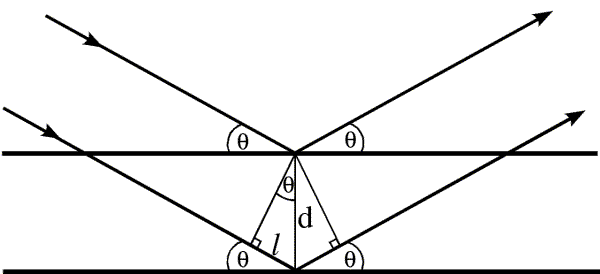# X-Ray Diffraction (XRD)

X-ray diffraction(XRD) is an important technique for determining the structures of crystals. The theory behind XRD is a result of pioneering works of the Braggs(father and son).

The main principle behind XRD is the diffraction of X-rays in specific directions by atoms in crystalline structure. Since the interplanar distances in crystals are of the order of Angstroms, therefore X-rays are the suitable choice, as the wavelength of the wave should be of the order of the slit size for the phenomenon of diffraction to take place.

Braggs suggested that the crystal structure can be considered as an array of atoms forming several planes. The X-rays on striking an atom will scatter in some direction. The scattered X-rays can interfere constructively if they are in phase or destructively if they are out of phase. Have a look at the following figure.The path difference between the two X-rays scattering from the two planes can be easily seen to be$2d\sin{\theta}$ where d is the interplanar spacing and$\theta$ is the incident angle.

For the waves to interfere constructively, they should satisfy the following relation$2d\sin{\theta}=n\lambda$

This is the Bragg’s Law.
Here,$\lambda$ is the wavelength of the X-ray beam. Therefore the path difference between the X-rays scattered from two planes should be an integer multiple of the wavelength.

Essentially, we consider the crystal to be a diffraction grating for an X-ray.

XRD pattern: A simple X-ray diffraction experimental setup requires the following: a radiation source, a sample crystal, and a detector. These are arranged as shown in the given figure.

The source gives off X-rays that strike the crystal at some angle$\theta$, and the detector detects the scattered radiation, at angle of$2\theta$ from the source. The X-rays hit the atoms in the crystal plane and scatter in different directions. For diffraction to occur, the scattered radiation must be coherent(in phase), therefore the scattering must be elastic. The angular position of the source is varied over the range of interest and correspondingly the detector, and the intensities of the scattered radiations are recorded and plotted versus$2\theta$. This plot of intensity of the scattered radiaiton vs$2\theta$ is known as an XRD pattern. (Alternatively, sample can be rotated.)The detector can be of several kinds. It can be a simple photographic plate or a sophisticated Geiger/Scintillation counter. The intensity of the highest peak is set to 100 and the rest of the peaks are scaled with respect to this. Then the peaks are mapped to a particular set of lattice planes given by the corresponding miller indices.
This is done by calculating the interplanar spacing(d) corresponding to a particular peak. And then using a relation between d and the (hkl) indices for different lattice types:
Cubic:$\frac{1}{d^2}=\frac{h^2+k^2+l^2}{a^2}$
Tetragonal:$\frac{1}{d^2}=\frac{h^2+k^2}{a^2}+\frac{l^2}{c^2}$
Hexagonal:$\frac{1}{d^2}=\frac{4}{3}\left(\frac{h^2+hk+k^2}{a^2}\right)+\frac{l^2}{c^2}$
Rhombohedral:$\frac{1}{d^{2}}=\frac{(h^{2}+k^{2}+l^{2})\sin ^{2}\alpha +2(hk+kl+hl)(\cos ^{2}\alpha -\cos \alpha )}{a^{2}(1-3\cos ^{2}\alpha +2\cos ^{3}\alpha )}$
Orthorhombic:$\frac{1}{d^2}=\frac{h^2}{a^2}+\frac{k^2}{b^2}+\frac{l^2}{c^2}$
Monoclinic:$\frac{1}{d^2}=\left(\frac{h^2}{a^2}+\frac{k^2\sin^2{\beta}}{b^2}+\frac{l^2}{c^2}-\frac{2hl\cos{\beta}}{ac}\right)\csc^2{\beta}$
Triclinic:$\frac{1}{d^2}=\frac{\frac{h^2}{a^2}\sin^{2}\alpha +\frac{k^2}{b^2}\sin^2\beta +\frac{l^2}{c^2}\sin^2\gamma +\frac{2kl}{bc}\cos \alpha +\frac {2hl}{ac}\cos \beta +\frac{2hk}{ab}\cos \gamma }{1-\cos^2\alpha -\cos^2\beta -\cos^2\gamma +2\cos \alpha \cos \beta \cos \gamma }$

Generally, a crystal would consist of many planes oriented in different directions. When X-rays strike the crystal at an angle$\theta$ to a particular plane, then if this angle, satisfies the Bragg’s law, then we would get an intensity peak corresponding to that particular plane in the XRD pattern. All the parallel planes contribute to a particular peak. If the incident angle of X-rays is changed to different values, then we may or may not get intensity peaks in the XRD pattern corresponding to the different planes depending on whether they satisfy the Bragg’s law or not.

The following are some of examples of the XRD pattern that one obtains:

Sometimes several diffraction orders are absent due to symmetries in the crystal. Consider the bcc lattice type. The peak corresponding to the (100) is missing in the XRD pattern. This is because at the Bragg angle for these planes, the atoms at the body center, diffract X-rays at 180 degrees out of phase relative to the atoms in the corners. As the number of atoms in the corners is equal to the atoms in the center, the diffracted beams get completely cancelled. Therefore, for a BCC cell, peaks corrsponding to (h+k+l)=odd are absent.## One thought on “X-Ray Diffraction (XRD)”

1. PLEASE PROVIDE TIO2 XRD PATTERN AND NAME OF UNIT CELL AND LATTICE COSTT. CALCULATION